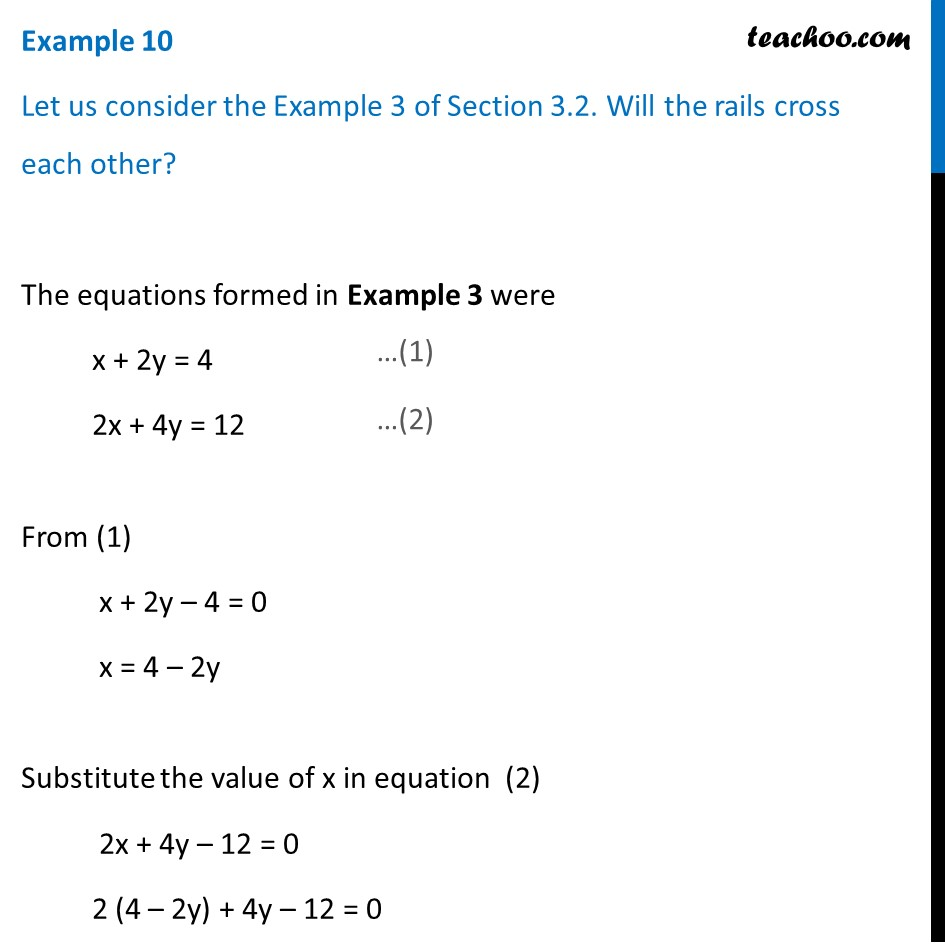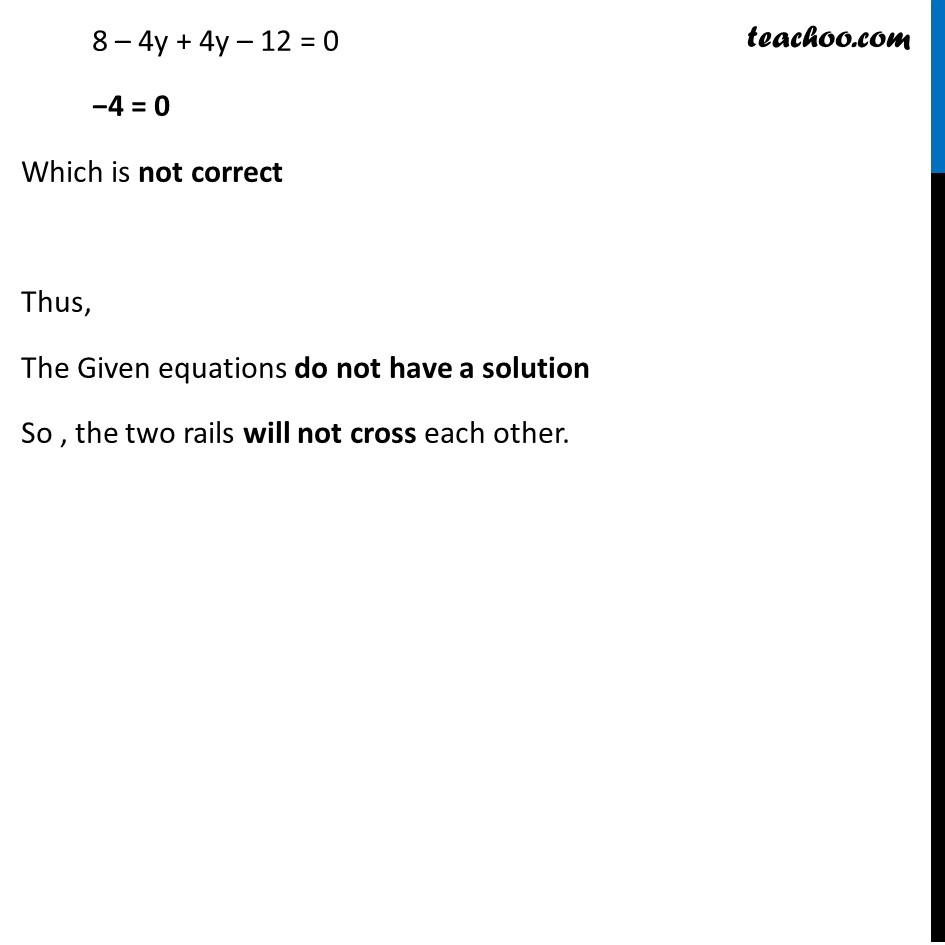Chapter 3 Class 10 Pair of Linear Equations in Two Variables

Class 10
Important Questions for Exam - Class 10Learn in your speed, with individual attention - Teachoo Maths 1-on-1 Class

### Transcript

Example 7 Let us consider the Example 3 of Section 3.2. Will the rails cross each other? The equations formed in Example 3 were x + 2y = 4 2x + 4y = 12 From (1) x + 2y – 4 = 0 x = 4 – 2y Substitute the value of x in equation (2) 2x + 4y – 12 = 0 2 (4 – 2y) + 4y – 12 = 0 8 – 4y + 4y – 12 = 0 −4 = 0 Which is not correct Thus, The Given equations do not have a solution So , the two rails will not cross each other.